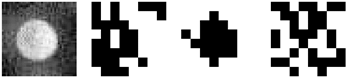# Template Matching¶

## Overview¶

Template matching is a technique where the objects are found across frames by using a matching algorithm that compares all possible objects, within a user specified search radius ($$r_{search}$$), and selects the object with the minimum difference. The algorithm matches objects across frames by comparing templates, which is a user selectable number of pixels centered on an object. These templates can be compared using one of several metrics including

• aHash – average image hashing

• pHash – perceptual image hashing

• dHash – difference image hashing

• SSE – sum of squared errors

• MSE – mean squared error

• L1 norm

• Linf norm

The algorithm is simple with no projection of where the object should be in the next frame. A user selectable search radius ($$r_{search}$$) can be used to limit the search area, improving performance of the algorithm. The algorithm steps are as follows:

Frame

Description of step

n

An object is found

n + 1

Within $$r_{search}$$, find the object with the closest Hamming distance for the image hashes or the smallest error metric.

n + i

Repeat the n+1 step until an object cannot be found within $$r_{search}$$ or the minimum distance is larger than the maximum difference for the image hashes or the smallest error metric is larger than the maximum difference for the other metrics.

This algorithm works well for tracking unique objects. Unfortunately for the granular systems typically investigated in gas-solid multiphase flow reactors, the particles are not unique enough to overcome changes in lighting and rotation to accurately match the same particle across multiple frames.

## Hashing Methods¶

Three of the matching techniques use image hashing. Image hashing attempts to create a unique low memory fingerprint of the object template by scaling the template down (typically to 8 x 8 pixels) and using a hashing algorithm to binarize the pixels. Currently implemented hashing algorithms include:

### dHash – difference image hashing¶

The average image hashing algorithm binarizes the pixels based on whether the left pixel is brighter than the right pixel.

### aHash – average image hashing¶

The average image hashing algorithm binarizes the pixels based on whether the pixel is brighter or darker than the average grayscale value.

### pHash – perceptual image hashing¶

The perceptual image hashing algorithm is more complicated than the others. The template is re-sized to 4 times the hash size (typically 32 x 32). A discrete cosine transform (DCT) is then applied to the grayscale matrix. The top-left hash size (typically 8x8) pixels, which represent the lowest frequencies in the picture, are used to calculate the resulting hash by comparing each pixel to the median value.

### Comparing hashes¶

The resulting hash can then be compared to other hashes by calculating the Hamming distance (which is the number of different bits). The smaller the Hamming distance, the more similar the hashes are. This technique is commonly used to efficiently perform reverse image searches.From left to right: example particle template, difference hash, average hash, and perceptual hash.